Question

# In an outdoor theatre environment where the source is emitting 10 watts of power how far...

In an outdoor theatre environment where the source is emitting 10 watts of power how far away must a patron be in order to be exposed to a sound intensity level of only 85dB?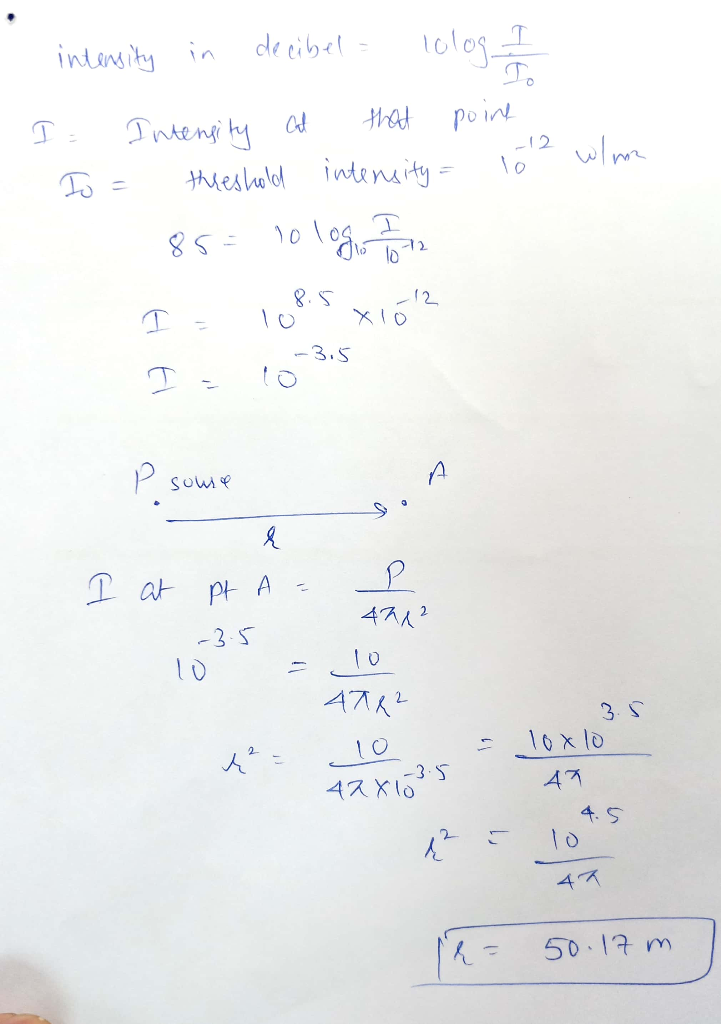#### Earn Coins

Coins can be redeemed for fabulous gifts.

Similar Homework Help Questions
• ### 0 A sound point source, situated 2.0 m above a brick floor, is emitting sound at...0 A sound point source, situated 2.0 m above a brick floor, is emitting sound at 4x10 watts. (a) Find the sound intensity level due to that source at a listening noint 3.5 m above the floor and 15 m away. (b) Find the sound intensity level at that point when the reflection from the floor is taken into account.

• ### 5. A point source emits a sound with an average power output of 80.0w. a) What...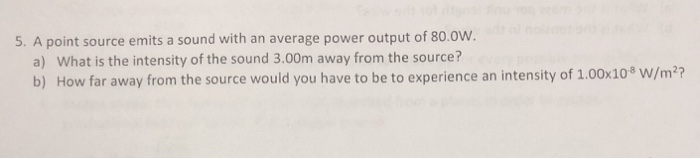5. A point source emits a sound with an average power output of 80.0w. a) What is the intensity of the sound 3.00m away from the source? b) How far away from the source would you have to be to experience an intensity of 1.00x108 W/m2?

• ### A point source emits sound waves with a power output of 100 watts. What is the...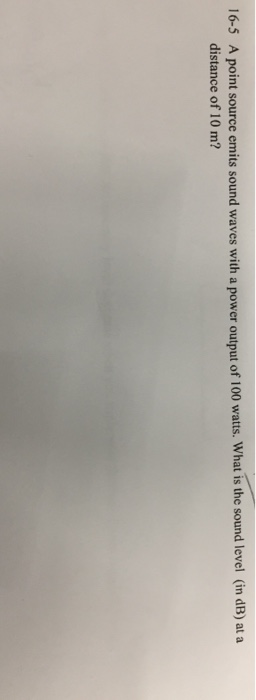A point source emits sound waves with a power output of 100 watts. What is the sound level (in dB) at a distance of 10 m? 16-5

• ### PHYSICS SELF TEST V 1. A source of sound emits 10.0 watts at the very centre...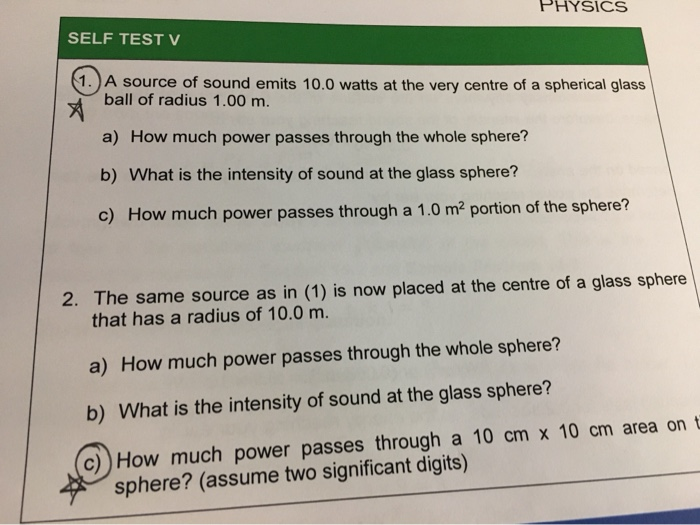PHYSICS SELF TEST V 1. A source of sound emits 10.0 watts at the very centre of a spherical glass ball of radius 1.00 m. a) How much power passes through the whole sphere? b) What is the intensity of sound at the glass sphere? c) How much power passes through a 1.0 m2 portion of the sphere? 2. The same source as in (1) is now placed at the centre of a glass sphere that has a radius of...

• ### Question 1 The power radiated by a lossless antenna is 10 watts.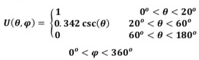Question 1 The power radiated by a lossless antenna is 10 watts. The directional characteristics of the antenna are represented by the radiation intensity of U = 2cos3θ watts/unit solid angle. Find the (a) Maximum power density at a distance of 1000 m (assume far-field distance) (b) Maximum directivity (c) Maximum gain Question 2 In target-search ground-mapping radars it is desirable to have echo power received from a target, of constant cross section, to be independent ofits range. For one such application, the desirable radiation intensity...

• ### 9. Sound levels are measured by the logarithmic decibel scale, named after Alexander Graham Bell. The sound level, L, corresponding to an intensity of I, in watts per square metre, is し= 10 log-, wh...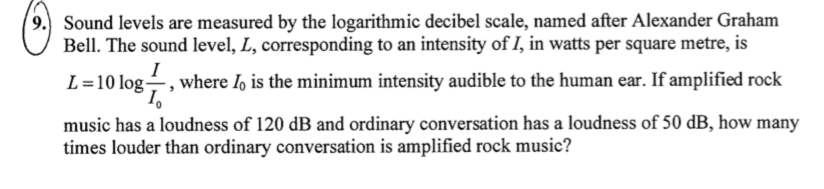9. Sound levels are measured by the logarithmic decibel scale, named after Alexander Graham Bell. The sound level, L, corresponding to an intensity of I, in watts per square metre, is し= 10 log-, where lo is the minimum intensity audible to the human ear. If amplified rock music has a loudness of 120 dB and ordinary conversation has a loudness of 50 dB, how many times louder than ordinary conversation is amplified rock music? 9. Sound levels are measured...

• ### Your friend is belching the alphabet. Where you are standing, a distance of 2 meters from...

Your friend is belching the alphabet. Where you are standing, a distance of 2 meters from your friend, the peak sound level of their belching is 68.2 dB. You would like to move far enough away from them that you won’t have to hear their belching. Assuming that the belch noises approximate spherical waves, how far should you stand from your friend in order for the peak intensity of the sound to be the threshold of hearing, 10^−12 W/m2?

• ### So i have the solution for this problem, but my questions is that when they were...

So i have the solution for this problem, but my questions is that when they were finding the sound intensity level where did they get the initial intensity? ( 1 × 10−12) A speaker with a power of 58 W is playing music. What is the sound intensity level of the music at a distance of 22 m? Assume that the sound can only be heard in front of the speaker and not behind it. Solution: In order to find...

• ### What was the power output of the speaker, assuming uniform spherical spreading of the sound and...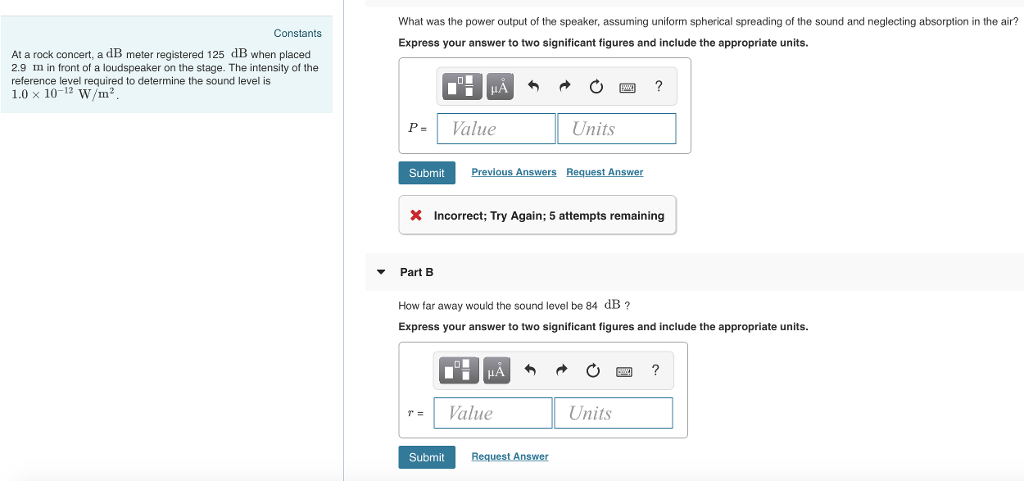What was the power output of the speaker, assuming uniform spherical spreading of the sound and neglecting absorption in the air? Express your answer to two significant figures and include the appropriate units. Constants At a rock concert, a dB meter registered 125 dB when placed 2.9 Im in front of a loudspeaker on the stage. The intensity of the reference level required to determine the sound level is 1.0 x 10-12 W/m? 勺 ? ' P-1 Value Units Submit...

• ### 27. Two musicians play and hold a note that has a Temp is 10° C frequency...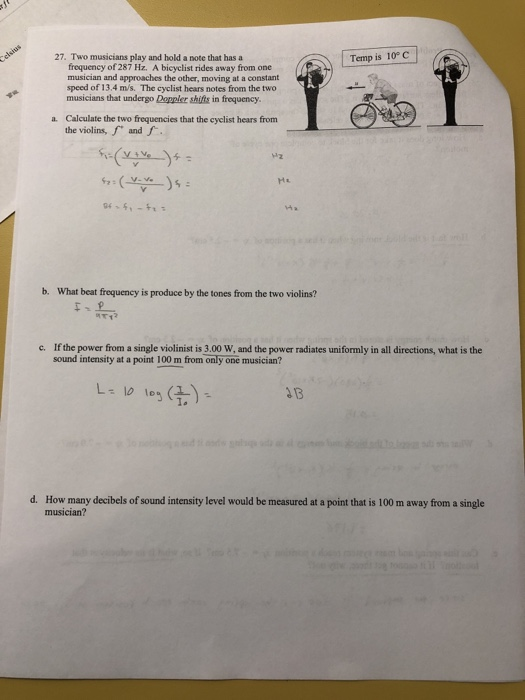27. Two musicians play and hold a note that has a Temp is 10° C frequency of 287 Hz. A bicyclist rides away from one musician and approaches the other, moving at a constant speed of 13.4 m/s? The cyclist hears notes from the two musicians that undergo Doppler shitits in frequency. Calculate the two frequencies that the cyclist hears from the violins, f and f a. 12 b. What beat frequency is produce by the tones from the two...